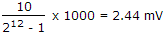# Electronics and Communication Engineering - Digital Electronics - Discussion

26.

A 12 bit ADC is used to convert analog voltage of 0 to 10 V into digital. The resolution is

 [A]. 2.44 mV [B]. 24.4 mV [C]. 1.2 V [D]. none of the above

Explanation:.

 Rajkumar said: (Aug 18, 2014) What is the formula used here?

 Dilip N said: (Feb 13, 2015) 10/(2^12 - 1) is 0.002442. When you multiply with 1000 we get 2.442? How come it is 2.44m?

 Hareesh Babu said: (Sep 4, 2015) But answer is given by mv that why multiply by 1000.

 Ansu said: (Aug 4, 2016) Because in question, it's given v.

 Sameer said: (Aug 21, 2016) But, the answer should be in the form of mV that's why he multplyed by 1000.

 Deepshikha said: (Jun 20, 2017) Formula used : R=V/(2^n)-1. Where R= resolution. V=voltage. N =bit used.

 Rishi Ram Panth said: (Jul 25, 2017) Thank you so much for all.

 Arun Shunmugam said: (Sep 7, 2017) Thank you all for explaining it.

 Deepak said: (Oct 19, 2017) Why 1000? Explain it.

 Faiyaz said: (Feb 6, 2018) @Deepak. Because option has given in mv.

 Akshata said: (Jun 21, 2019) @All. Why you are multiply by 1000. It is not necessary; 10/2^12-1 = 2.44mv. Formula =E(v)/2^n-1 (n=no of bits).